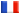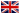# Séminaire de Cryptographie

## Robert Carls### Serre-Tate theory and converging AGM-sequences

Let A be an abelian variety over a finite field. Liftable endomorphisms of A act on the deformation space. In the ordinary case there's a canonical way of lifting Frobenius. We will show, that the action of Frobenius has a unique fixpoint, the canonical lift. A proof will be given in terms of Barsotti-Tate groups using the Serre-Tate theorem. Drinfeld's proof of this theorem will be sketched (see ). It will be explained how to make the above action explicit for elliptic curves. In characterictic 2 one can describe the action by the AGM (arithmetic geometric mean) sequence.

References :

 N.Katz: Serre-Tate local moduli, in 'surfaces algebriques', Springer lecture notes 868, 1981

 R.Carls: in prep., http://www.math.leidenuniv.nl/~carls/extract.ps